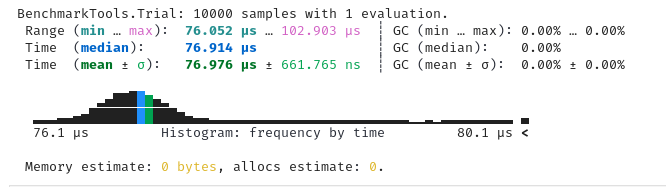# Need help understanding the allocations

Consider the following code

``````using BenchmarkTools
using Random

f(p, xm, x) = -x^2 / ((p - xm*x)^2 + (p - xm*x)^2)
g(p, xm, x) = -x^2 / ((p - xm)^2 + (p - xm)^2)
integralf(p, xm) = quadgk(x -> f(p, xm, x), 0.0, 1.0)
integralg(p, xm) = quadgk(x -> g(p, xm, x), 0.0, 1.0)

function test(p, xm, integral)
s = 0.0
for i in 1:1000
rand!(p)
rand!(xm)
res, err = integral(p, xm)
s += res
end
return s
end
p  = zeros(Float64, 2)
xm = zeros(Float64, 2)
test(p, xm, integralf)
test(p, xm, integralg)
``````

When I run the benchmarks, `integralf` is allocating, whereas `integralg` ( which only has `x^2` in it) is not, which I do not understand.

`@benchmark test(\$p, \$xm, \$integralf)`

`@benchmark test(\$p, \$xm, \$integralg)`Can someone help me understanding what is going on?

EDIT: I think it is related to the fact that there are `1/(a-x)` kind of terms in the integrand, as shown by allocation profiler. With the division replaced by multiplication in `f(p, xm, x)`, it vanishes.

One thing is that you better define your test function like this:

``````julia> function test(p, xm, integral::F) where {F<:Function}
s = 0.0
for i in 1:1000
rand!(p)
rand!(xm)
res, err = integral(p, xm)
s += res
end
return s
end
``````

because Julia by default may not specialize to function arguments, and this can cause allocations (although here you are using the function, so it should).

That being said, here this does not solve the problem. And since the direct call to each integral is not allocating, I think you reached some weird bug.

I believe the allocations come from the algorithm performing further subdivisions in the case of f, while it can early return for g, because the error estimate is sufficiently small already.

Step through it with Debugger and you’ll see what I mean.

2 Likes

Or use staticarrays

Running the integral functions outside the loop doesn’t allocate anything (can that be dependent on the random point given?)

It can be different. Quadgk is looking to see if the error bound is being met and that will be parameter dependent.

1 Like

Indeed, sometimes it allocates, sometimes it doesn’t:

``````julia> @btime integralf(\$(rand(2)), \$(rand(2)))
55.014 ns (0 allocations: 0 bytes)
(-0.3320182264630196, 0.0)

julia> @btime integralf(\$(rand(2)), \$(rand(2)))
307.376 ns (2 allocations: 368 bytes)
(-2.1198229226184617, 9.467969463994308e-10)

julia> @btime integralf(\$(rand(2)), \$(rand(2)))
517.234 ns (2 allocations: 368 bytes)
(-4.848361174937609, 1.5858105731347827e-8)

julia> @btime integralf(\$(rand(2)), \$(rand(2)))
627.907 ns (2 allocations: 368 bytes)
(-18.88726883755866, 1.4078685466045737e-7)

julia> @btime integralf(\$(rand(2)), \$(rand(2)))
57.118 ns (0 allocations: 0 bytes)
(-0.4933392928396032, 7.264673307361136e-10)
``````

Does not work here:

``````julia> @btime integralf(\$(rand(SVector{2,Float64})), \$(rand(SVector{2,Float64})))
307.844 ns (2 allocations: 368 bytes)
(-2.7657212478611743, 4.964859356970663e-10)
``````

Indeed as @skleinbo and @Oscar_Smith pointed out, it is because quadgk is using adaptive methods to meet the error bounds on the integral. The allocation profiler output also says so.

Changing the `rtol` parameter for `quadgk` changes the total allocations. Higher the `rtol`, lower the allocations for `integralf`.
`integralf(p, xm) = quadgk(x -> f(p, xm, x), 0.0, 1.0, rtol=1.e-4)`
`rtol=1.e-4 : Memory estimate: 112.02 KiB, allocs estimate: 609.`
`rtol=1.e-2 : Memory estimate: 42.19 KiB, allocs estimate: 222.`

Is there a way to preallocate memory that quadgk can reuse? The integrand and the limits do not change, only the parameters `p` and `xm` do. I tried `segbuf` option but it increases the allocations.

Yes, you can pass that via the `segbuf` keyword. See the docstrings of `quadgk` and `alloc_segbuf` for how to do it.

However, I presume the algorithm is subdividing a lot because the integrand has a singularity, which may not be what you intend.